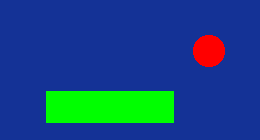# Hungry Serpent

Tweet blitz3d blitzbasic code-archives
(Posted 2 months ago)

A simple snake clone written in Blitz3D.

Hungry Serpent is just a very basic Snake-Clone with simple, computer generated graphics. The game does not use any external ressources.

Author: SparkFountain

You move a green snake over the screen and navigate it to the red apples. Every time the snake gulps an apple, it grows a bit and moves faster. After some time, massive gray rocks will appear which you have to avoid. If the snake hits a border or rock or bites itself, the game is over.``````;HUNGRY SERPENT
;==============

AppTitle("Hungry Serpent")

;Screen resolution (in square fields, each square 32x32 pixels)
Global screenX = 25
Global screenY = 20

Graphics(screenX*32,screenY*32,16,2)
SetBuffer(BackBuffer())
Global frameTimer = CreateTimer(60)
SeedRnd(MilliSecs())

ClsColor(20,50,150)
HidePointer()

;GLOBALS
Global snakeX
Global snakeY
Global snakeLength
Global numberOfApples
Global score

Global lastMillis
Global waitSnakeTimer

Global language\$ = "en"

;GUI FONT
SetFont(guiFont)

;first time when game starts
InitGame()

;=== MAIN LOOP
Repeat
WaitTimer(frameTimer)
Cls()

DrawApple()
DrawRock()
MoveSnake()
DrawSnake()
SnakeCollides()
ToggleLanguage()
GUI()

Flip(0)
Until(KeyHit(1))
End()
;=== END OF MAIN LOOP

Function InitGame()
FlushKeys()

;delete old snake, apples, rocks and wayscore
Local s.Snake
For s.Snake = Each Snake
Delete s
Next

Local a.Apple
For a.Apple = Each Apple
Delete a
Next

Local r.Rock
For r.Rock = Each Rock
Delete r
Next

Local w.Waypoint
For w.Waypoint = Each Waypoint
Delete w
Next

;reset values
snakeX = screenX-4
snakeY = screenY/2
snakeLength = 0

lastMillis = 0
waitSnakeTimer = 200

numberOfApples = 0
score = 0

s.Snake = New Snake
s\x = snakeX
s\y = snakeY
s\direction = "left"
snakeLength = snakeLength+1

;at the beginning, snake consists of 4 parts (1 head, 3 tail)
Local i
For i=0 To 2
ElongateSnake()
snakeLength = snakeLength+1
Next

;create first apple
CreateApples()
End Function

Function GetValidCoordinates\$(forElement\$)
Local validCoordinates
Local x,y
Local s.Snake, a.Apple, r.Rock
Repeat
validCoordinates = True
x = Rnd(1,screenY-1)
y = Rnd(1,screenY-1)

;don't create the object where the snake already is
For s.Snake = Each Snake
If(s\x=x And s\y=y) Then validCoordinates = False
Next

;if apple: don't create where a rock is
If(forElement\$ = "apple") Then
For r.Rock = Each Rock
If(r\x=x And r\y=y) Then validCoordinates = False
Next
EndIf

;if rock: don't create where an apple is
If(forElement\$ = "apple") Then
For a.Apple = Each Apple
If(a\x=x And a\y=y) Then validCoordinates = False
Next
EndIf
Until(validCoordinates=True)

Return(x+","+y)
End Function

Function CreateApples()
Local i
For i=1 To snakeLength/5+1
Local a.Apple = New Apple
Local coordinates\$ = GetValidCoordinates("apple")
a\x = Int(Left(coordinates\$,Instr(coordinates\$,",")))
a\y = Int(Mid(coordinates\$,Instr(coordinates\$,",")+1))
numberOfApples = numberOfApples+1
Next
End Function

Function DrawApple()
SetColor("apple")
Local a.Apple
For a.Apple = Each Apple
Oval(a\x*32,a\y*32,32,32,1)
Next
End Function

Function CreateRock()
Local coordinates\$ = GetValidCoordinates("rock")
Local r.Rock = New Rock
r\x = Int(Left(coordinates\$,Instr(coordinates\$,",")))
r\y = Int(Mid(coordinates\$,Instr(coordinates\$,",")+1))
End Function

Function DrawRock()
SetColor("rock")
Local r.Rock
For r.Rock = Each Rock
Oval(r\x*32,r\y*32,32,32,1)
Next
End Function

Function MoveSnake()
If (MilliSecs()-lastMillis) > waitSnakeTimer Then
lastMillis = MilliSecs()    ;reset timer
If(KeyHit(200))
Local w.Waypoint
w.Waypoint = New Waypoint
w\passed = 1
w\direction\$ = "up"
EndIf
ElseIf(KeyHit(208))
w.Waypoint = New Waypoint
w\passed = 1
w\direction\$ = "down"
EndIf
ElseIf(KeyHit(203))
w.Waypoint = New Waypoint
w\passed = 1
w\direction\$ = "left"
EndIf
ElseIf(KeyHit(205))
w.Waypoint = New Waypoint
w\passed = 1
w\direction\$ = "right"
EndIf
EndIf

Local s.Snake
For s.Snake = Each Snake
Select s\direction
Case "up"
s\y=s\y-1
Case "down"
s\y=s\y+1
Case "left"
s\x=s\x-1
Case "right"
s\x=s\x+1
End Select
Next

CheckWayscore()
EndIf
End Function

Function ElongateSnake()
;get tail of snake to add a new part behind
Local tail.Snake = Last Snake

Local s.Snake = New Snake
Select tail\direction
Case "up"
s\x = tail\x
s\y = tail\y+1
s\direction\$ = "up"
Case "down"
s\x = tail\x
s\y = tail\y-1
s\direction\$ = "down"
Case "left"
s\x = tail\x+1
s\y = tail\y
s\direction\$ = "left"
Case "right"
s\x = tail\x-1
s\y = tail\y
s\direction\$ = "right"
End Select
End Function

Function DrawSnake()
SetColor("snake")
Local s.Snake
For s.Snake = Each Snake
Rect(s\x*32,s\y*32,32,32,1)
Next
End Function

Function SnakeCollides()
Local s.Snake
For s.Snake = Each Snake
;bite yourself
Local s2.Snake
For s2.Snake = Each Snake
If (s <> s2 And s\x=s2\x And s\y=s2\y) Then
If(language="en") Then
ShowWarning("You have bitten yourself!")
Else
ShowWarning("Du hast dich selbst gebissen!")
EndIf
InitGame()
Return  ;quit current loop
EndIf
Next
Next

;eat an apple
Local a.Apple
For a.Apple = Each Apple
snakeLength=snakeLength+1
score=score+100
Delete a
numberOfApples = numberOfApples-1
Local r.Rock
For r.Rock = Each Rock
Delete r
Next
ElongateSnake()
If(numberOfApples=0) Then CreateApples()
If(snakeLength>4) Then
Local i
For i=0 To snakeLength/4
CreateRock()
Next
EndIf
waitSnakeTimer=waitSnakeTimer-1 ;decreasing value => lower interval
EndIf
Next

;collide with a rock
For r.Rock = Each Rock
If(language="en") Then
ShowWarning("You hit a massive rock!")
Else
ShowWarning("Du hast einen Felsen gerammt!")
EndIf
InitGame()
Return  ;quit current loop
EndIf
Next

If(language="en") Then
ShowWarning("You touched the border!")
Else
ShowWarning("Du hast den Rand berührt!")
EndIf
InitGame()
Return  ;quit current loop
EndIf
End Function

Function CheckWayscore()
Local s.Snake
For s.Snake = Each Snake
Local w.Waypoint
For w.Waypoint = Each Waypoint
If (s\x=w\x And s\y=w\y) Then
s\direction\$ = w\direction\$
w\passed=w\passed+1
EndIf

If (w\passed = snakeLength) Then
Delete w
EndIf
Next
Next
End Function

Function ShowWarning(message\$)
Local guiScore\$

;draw a nice box around the warning message
SetColor("warning box")
Rect(screenX*32/2-StringWidth(message\$)/2-25,screenY*32/2-50,StringWidth(message\$)+50,100)
SetColor("warning")
Text(screenX*32/2,screenY*32/2-25,message\$,screenX*32/2,screenY*32/2-25)
If(language\$="en") Then
ElseIf(language="de") Then
guiScore\$ = "Deine Punkte: "
EndIf
Text(screenX*32/2,screenY*32/2+25,guiScore\$+score,screenX*32/2,screenY*32/2+25)
Flip(0)
Delay(2500)
End Function

Function ToggleLanguage()
If(KeyHit(18)) Then
language\$ = "en"
ElseIf(KeyHit(32)) Then
language\$ = "de"
EndIf
End Function

Function SetColor(c\$)
Select c\$
Case "apple"
Color(255,0,0)
Case "rock"
Color(128,128,128)
Case "snake"
Color(0,255,0)
Case "score"
Color(255,255,0)
Case "length"
Color(255,128,128)
Case "warning"
Color(128,128,0)
Case "warning box"
Color(30,30,100)
End Select
End Function

Function GUI()
Local guiScore\$, guiLength\$
If(language\$="en") Then
guiScore\$ = "Score: "
guiLength\$ = "Length of snake: "
ElseIf(language="de") Then
guiScore\$ = "Punkte: "
guiLength\$ = "Länge der Schlange: "
EndIf

SetColor("score")
Text((screenX*32/2),10,guiScore\$+score,(screenX*32/2))
SetColor("length")
Text ((screenX*32/2),40,guiLength\$+snakeLength,(screenX*32/2))
End Function

Type Apple
Field x,y
End Type

Type Rock
Field x,y
End Type

Type Snake
Field x,y
Field direction\$
End Type

Type Waypoint
Field x,y
Field direction\$
Field passed    ;how often this waypoint has already been passed by the snake
End Type
;~IDEal Editor Parameters:
;~C#Blitz3D``````